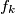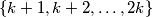### IMO Shortlist 1994 problem N5

Kvaliteta:
Avg: 0,0
Težina:
Avg: 8,0
For any positive integer$k$, let$f_k$ be the number of elements in the set$\{ k + 1, k + 2, \ldots, 2k\}$ whose base 2 representation contains exactly three 1s.

(a) Prove that for any positive integer$m$, there exists at least one positive integer$k$ such that$f(k) = m$.

(b) Determine all positive integers$m$ for which there exists exactly one$k$ with$f(k) = m$.
Izvor: Međunarodna matematička olimpijada, shortlist 1994